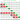# Insertion Sort Algorithm

May 11, 2019Its a kind of incremental insertion technique, where the algorithm build up sorting by first sorting n-1 items.

Or, we can say that we pick an element on n-1 items, and insert in a position where all items on the left side are less than or equal to this number.

## Insertion Sort Algorithm

The algorithm works in two loops, where outer most loop iterate over complete array. To be more precise, We iterate from index-1 to end of array. Leaving index-0 to be used by inner loop.

In inner loop, the loop variable takes the index from outer loop index variable. It takes a backup of the value present on that index, and go backward toward 0-index. It shift previous elements to next position unless they are greater than the value for which we have taken backup.

In short, inner loop tries to sort the n-1 part of array by shifting any bigger element to right. And, insert the element to the final position.

See the code here:

``````public void sort(int[] arr) {
int len = arr.length;
for (int i=1; i<len; i++) {
int key = arr[i];
int j = i-1;
while (j >= 0 && key < arr[j]) {
arr[j+1] = arr[j];
j--;
}

arr[j+1] = key;
}
}``````

## Graphical Example## Key Points

• It does not use extra memory. And, is in-place algorithm.
• Its stable, as it does not change order of same value elements.
• It is more efficient in practice than Bubble Sort and Selection Sort
• It is well suited for small data sets.

## Runtime complexity

The algorithm runs on O(n^2) in worst case.